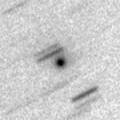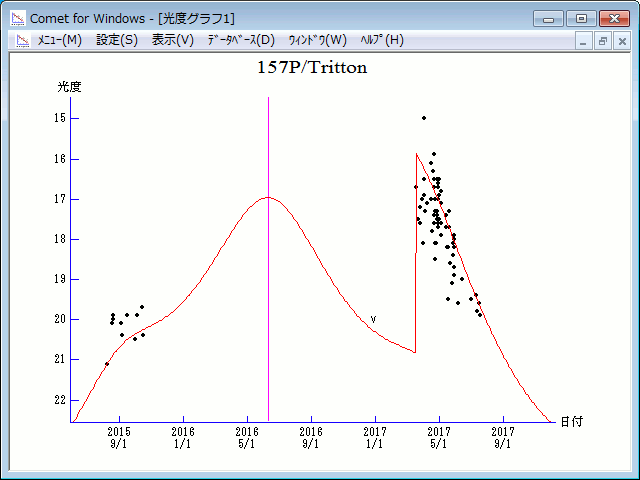# \$B%H%j%H%sWB@1(B

157P/Tritton (2016)###\$B###\$B50F;MWAG(B

```Epoch 2016 June 21.0 TT = JDT 2457560.5
T 2016 June 10.38711 TT                                 MPCW
q   1.3580788            (2000.0)            P               Q
n   0.15663513     Peri.  148.89767     +0.01563404     -0.99382885
a   3.4083428      Node   299.99958     +0.88934342     +0.06401726
e   0.6015428      Incl.    7.28502     +0.45697250     -0.09058699
P   6.29
From 1118 observations 2003 Oct. 6-2017 May 5, mean residual 0".8.
Nongravitational parameters A1 = +0.56, A2 = +0.0218.
```

###\$B8wEYJQ2=(B

```        m1 = 13.5 + 5 log\$B&\$(B + 12.0 log r  [   ,280]  (              \$B!A(B2017\$BG/(B 3\$B7n(B17\$BF|(B)
m1 =  0.0 + 5 log\$B&\$(B + 30   log r  [280,   ]  (2017\$BG/(B 3\$B7n(B17\$BF|!A(B              )
```##### \$B50F;MWAG\$O(BM.P.E.C. 2017-J52\$B\$K7G:\\$5\$l\$?\$b\$N\$G\$9!#(B \$B8wEY%0%i%U\$O(BComet for Windows\$B\$G:n@.\$7\$?\$b\$N\$G\$9!#(B